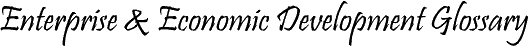calculation of a net figure from the original cost (base price) of an asset (fixed asset), taking into account certain items such as those that are tax-related, for example, the addition of capital expenditure and the deduction of depreciation allowances. One source for an adjusted basis calculator is www.ajdesigner.com/php_ab/adjusted_basis.php.

Wikipedia offers more information and shows how to calculate the net cost of an asset.

related: asset; asset management; adjusted cost basis (ACB); real estate; facilities

go back

FYI: definition of adjusted basis less depreciation define adjusted cost basis what does acb stand for in relation to adjusted basis definition of adjusted cost definition of base cost definition of base price definition of fixed asset define assets define property asset definition of capital expenditure definition of capital expenditures definition of depreciation allowances
define acb acronym define basis adjustment define adjusted cost define base cost define base price define fixed asset define asset define property assets define capital expenditure define capital expenditures define depreciation allowances